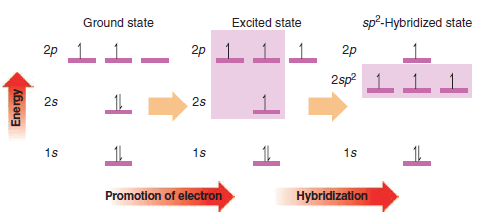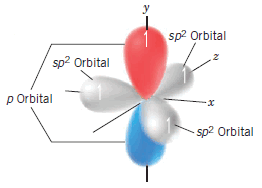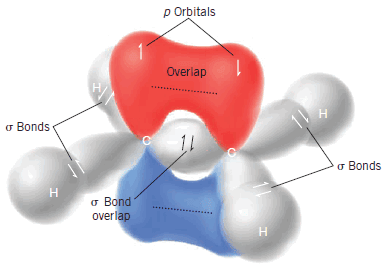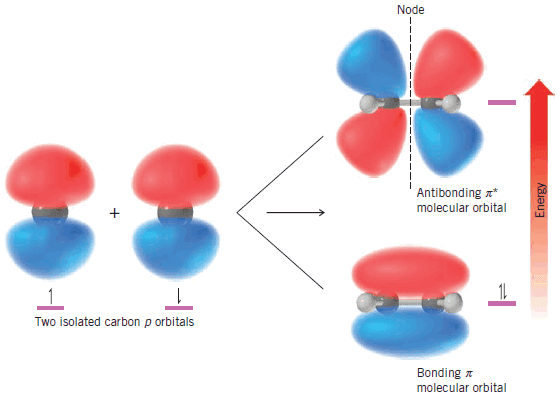## Breaking News

#### The Structure of Ethene (Ethylene): sp2 Hybridization

** The carbon atoms of many of the molecules that we have considered so far have used their four valence electrons to form four single covalent (sigma) bonds to four other atoms.

** We find, however, that many important organic compounds exist in which carbon atoms share more than two electrons with another atom. In molecules of these compounds some bonds that are formed are multiple covalent bonds. When two carbon atoms share two pairs of electrons, for example, the result is a carbon–carbon double bond:

** Hydrocarbons whose molecules contain a carbon–carbon double bond are called alkenes. Ethene (C2H4) and propene (C3H6) are both alkenes. Ethene is also called ethylene, and propene is sometimes called propylene.

** In ethene the only carbon–carbon bond is a double bond. Propene has one carbon–carbon single bond and one carbon–carbon double bond.

** The spatial arrangement of the atoms of alkenes is different from that of alkanes. The six atoms of ethene are coplanar, and the arrangement of atoms around each carbon atom is triangular (Fig.1).

** Carbon–carbon double bonds are comprised of sp2-hybridized carbon atoms.

** The mathematical mixing of orbitals that furnish the sp2 orbitals for our model can be visualized in the way shown in Fig. 2.Fig (2)

The 2s orbital is mathematically mixed (or hybridized) with two of the 2p orbitals. (The hybridization procedure applies only to the orbitals, not to the electrons.) One 2p orbital is left unhybridized. One electron is then placed in each of the sp2 hybrid orbitals and one electron remains in the 2p orbital.

** The three sp2 orbitals that result from hybridization are directed toward the corners of a regular triangle (with angles of 120o between them). The carbon p orbital that is not hybridized is perpendicular to the plane of the triangle formed by the hybrid sp2 orbitals (Fig. 3).Figure (3)

** In our model for ethane (Fig. 4) we see the following:Figure (4)

(l) Two sp2-hybridized carbon atoms form a sigma (σ) bond between them by overlap of one sp2 orbital from each carbon. The remaining carbon sp2 orbitals form σ bonds to four hydrogens through overlap with the hydrogen 1s orbitals. These five σ bonds account for 10 of the 12 valence electrons contributed by the two carbons and four hydrogens, and comprise the σ-bond framework of the molecule.

(2) The remaining two bonding electrons are each located in an unhybridized p orbital of each carbon. Sideways overlap of these p orbitals and sharing of the two electrons between the carbons leads to a pi (π) bond. The overlap of these orbitals is shown schematically in Fig. 5.Figure (5)

** The bond angles that we would predict on the basis of sp2-hybridized carbon atoms (120o all around) are quite close to the bond angles that are actually found (Fig. 1).

** We can better visualize how these p orbitals interact with each other if we view a structure showing calculated molecular orbitals for ethene (Fig. 1.25). We see that the parallel p orbitals overlap above and below the plane of the s framework.

** Note the difference in shape of the bonding molecular orbital of a π bond as contrasted to that of a σ bond. A σ bond has cylindrical symmetry about a line connecting the two bonded nuclei. A π bond has a nodal plane passing through the two bonded nuclei and between the π molecular orbital lobes.

** When two p atomic orbitals combine to form a π bond, two π molecular orbital molecular orbitals form: one is a bonding molecular orbital and the other is an antibonding molecular orbital.

** The bonding π molecular orbital results when p-orbital lobes of like signs overlap; the antibonding π molecular orbital results when opposite signs overlap (Fig. 6).Figure (6)

** The bonding π orbital is the lower energy orbital and contains both p electrons (with opposite spins) in the ground state of the molecule. The region of greatest probability of finding the electrons in the bonding π orbital is a region generally situated above and below the plane of the σ-bond framework between the two carbon atoms. The antibonding π* orbital is of higher energy, and it is not occupied by electrons when the molecule is in the ground state. It can become occupied, however, if the molecule absorbs light of the right frequency and an electron is promoted from the lower energy level to the higher one. The antibonding π* orbital has a nodal plane between the two carbon atoms.

** To summarize, a carbon–carbon double bond consists of one σ bond and one π bond.

** The σ bond results from two sp2 orbitals overlapping end to end and is symmetrical about an axis linking the two carbon atoms. The π bond results from a sideways overlap of two p orbitals; it has a nodal plane like a π orbital. In the ground state the electrons of the π bond are located between the two carbon atoms but generally above and below the plane of the σ-bond framework.

** Electrons of the π bond have greater energy than electrons of the σ bond. The relative energies of the σ and π molecular orbitals (with the electrons in the ground state) are shown in the margin diagram. The σ* orbital is the antibonding sigma orbital.

#### Restricted Rotation and the Double Bond

** The σ–π model for the carbon–carbon double bond also accounts for an important property of the double bond:

** There is a large energy barrier to rotation associated with groups joined by a double bond.

** Maximum overlap between the p orbitals of a π bond occurs when the axes of the p orbitals
are exactly parallel.

** Rotating one carbon of the double bond 90o (Fig. 7) breaks the π bond, for then the axes of the p orbitals are perpendicular and there is no net overlap between them.

** Estimates based on thermochemical calculations indicate that the strength of the p bond is 264 kJ mol-1. This, then, is the barrier to rotation of the double bond. It is markedly higher than the rotational barrier of groups joined by carbon–carbon single bonds (13–26 kJ mol-1).

** While groups joined by single bonds rotate relatively freely at room temperature, those joined by double bonds do not.

#### Cis–Trans Isomerism

** Restricted rotation of groups joined by a double bond causes a new type of isomerism that we illustrate with the two dichloroethenes written as the following structures:

** These two compounds are isomers; they are different compounds that have the same molecular formula.

** We can tell that they are different compounds by trying to place a model of one compound on a model of the other so that all parts coincide, that is, to try to superpose one on the other. We find that it cannot be done. Had one been superposable on the other, all parts of one model would correspond in three dimensions exactly with the other model. (The notion of superposition is different from simply superimposing one thing on another. The latter means only to lay one on the other without the necessary condition that all parts coincide.)

** We indicate that they are different isomers by attaching the prefix cis or trans to their names (cis, Latin: on this side; trans, Latin: across).

** cis-1,2-Dichloroethene and trans-1,2-dichloroethene are not constitutional isomers because the connectivity of the atoms is the same in each. The two compounds differ only in the arrangement of their atoms in space. Isomers of this kind are classified formally as stereoisomers, but often they are called simply cis–trans isomers.

** The structural requirements for cis–trans isomerism will become clear if we consider a few additional examples. 1,1-Dichloroethene and 1,1,2-trichloroethene do not show this type of isomerism.

** 1,2-Difluoroethene and 1,2-dichloro-1,2-difluoroethene do exist as cis–trans isomers.

** Notice that we designate the isomer with two identical groups on the same side as being cis:

** Clearly, then, cis–trans isomerism of this type is not possible if one carbon atom of the double bond bears two identical groups.

#### Solved Problem

Write structures of all the isomers of C2H5F.